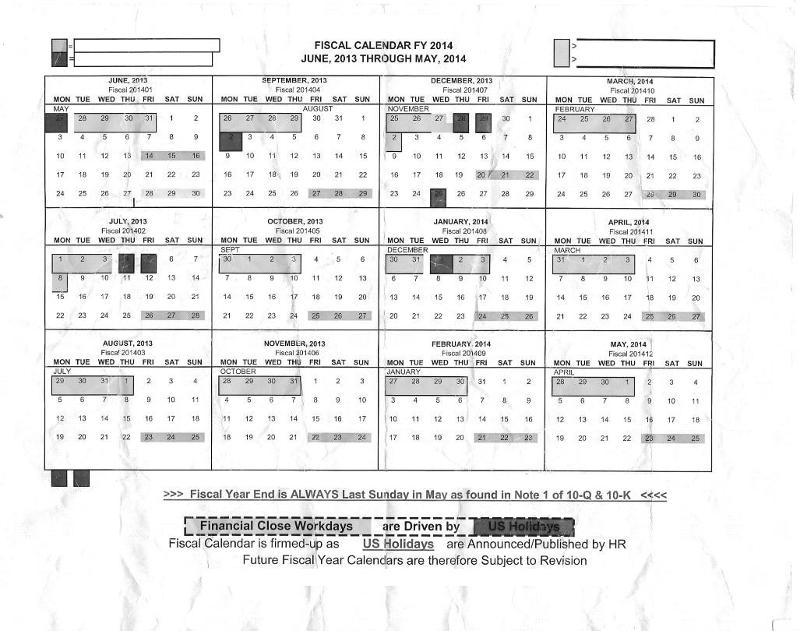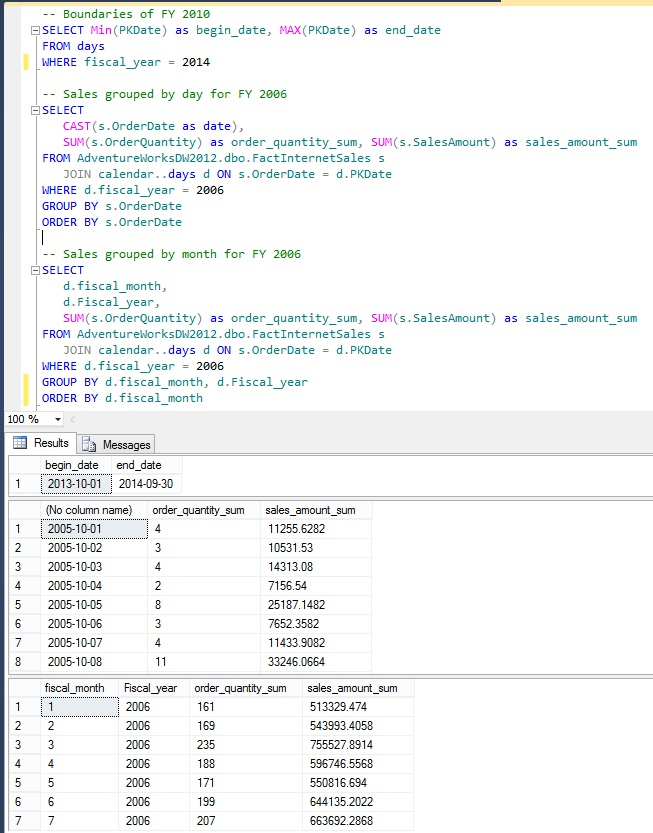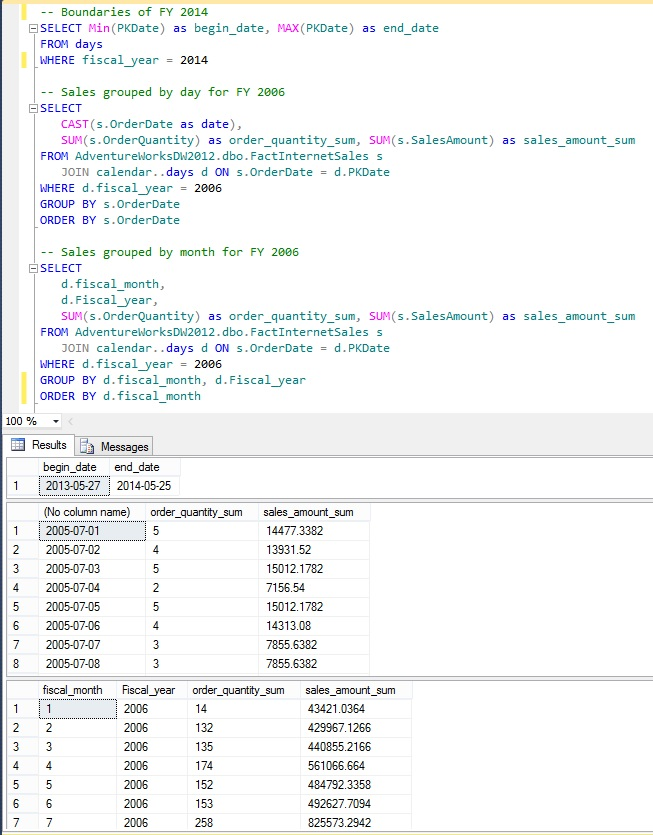# SQL Server Calendar Table:  Fiscal Years

Published on
13,583 Points
7,183 Views
4 Endorsements
Almost every company measures results in some kind of year whether it be calendar year or some other definition known as a Fiscal Year.  This article shows how to build on the SQL Server Calendar Table article to handle the Fiscal Year.
This is third in a series of articles dealing with table-based calendars in SQL Server.   Before executing any code go to the SQL Server Calendar Table article, scroll to the bottom, copy the complete T-SQL code, open up SQL Server Management Studio ( SSMS ), paste into a new query editor window, and execute.

Previous articles in the series
#1  SQL Server Calendar Table - Downloadable code to build the table.
#2  SQL Server Calendar Table - Tips and Tricks - Stored Procedures and Functions to handle querying for ranges of days based on user-defined criteria such as start date, previous or next days, include the start date, and return rows.

Summary
After executing the SQL Server T-SQL code in these articles and modifying to meet your needs you should be able to write custom code that uses fiscal time periods by JOINing your tables to the days table ON {your table.date column} = days.PKDate, and referencing the appropriate fiscal_whatever columns.

You will no longer have to write expressions in every query, and no longer need to store accounting month / week / whatever values in production tables unless needed for other reasons.

In Scope
• Introduction to Fiscal Year
• Easy:  Fiscal Years where Month Boundaries are the same as Calendar Year
• Hard:  4-4-5 Fiscal Year (can also be 4-5-4 or 5-4-4 but 4-4-5 is the commonly used term) or Broadcast Calendar

## Introduction to Fiscal Year

Calendar years run January through December, months run from day 1 to days from 28 to 31, and weeks have different definitions such as starting on Sunday or Monday based on location.   Businesses are not obligated to run their accounting calendars with these definitions, and will use different definitions to more accurately reflect their business cycle.  For example, many schools begin their fiscal year at the beginning of summer break, and many agriculture companies begin their fiscal year before planting starts.

The below image is an example from a previous client of a custom fiscal year

• The year begins the day after the last Sunday in May
• Months are defined as 5-4-4, where the first month has five weeks, the second and third months have four weeks, repeating through all twelve months.   This equates to ( 5 + 4 + 4 ) * 7 = 364 days, meaning every fourth year or so there is a 'leap day', which I'll not deal with in this article.
• Weeks begin on Mondays## Easy:  Fiscal Years where Month Boundaries are the same as Calendar Year

Reminder:  Before executing any code go to the SQL Server Calendar Table article, scroll to the bottom, copy the complete T-SQL code, open up SSMS, paste into a new query editor window, and execute.

To enable fiscal handling first create columns to hold the values for fiscal year, month, week, and day.

``````ALTER TABLE days
fiscal_year smallint,
fiscal_month smallint,
fiscal_week_in_month tinyint,
fiscal_week_in_year tinyint,
fiscal_day_in_month tinyint,
fiscal_day_in_year tinyint
``````
Let's populate them with an easy example:  A fiscal year that runs October 1 to September 30.  October is considered month #1, November is month #2, to September is month #12.   Each month has the same days as the calendar month, 1 through 28-31.

Set the fiscal year and month values...
``````-- The fiscal year is the same calendar year as the last nine months
UPDATE days
SET fiscal_year =
CASE WHEN calendar_month IN (10, 11, 12) THEN calendar_year + 1
ELSE calendar_year END

UPDATE days
SET fiscal_month = CASE calendar_month
WHEN 10 THEN 1 WHEN 11 THEN 2 WHEN 12 THEN 3
WHEN 1 THEN 4 WHEN 2 THEN 5 WHEN 3 THEN 6
WHEN 4 THEN 7 WHEN 5 THEN 8 WHEN 6 THEN 9
WHEN 7 THEN 10 WHEN 8 THEN 11 WHEN 9 THEN 12 END
``````
Now you can query these columns...

##Hard:  4-4-5 Fiscal Year

Let's build a calendar table based on the image at the top of this article.
This will require a nested WHILE loops:

• Identify the last day of the previous year, defined as the last Sunday in May.
• Use a WHILE loop to loop through each year, and a number of variables as counters ...
• Use a WHILE loop to loop through each month, handling 4 or 5 weeks, updating columns fiscal_year and fiscal_month, and a Common Table Expression ( CTE ) to update fiscal_day_in_month
• Use a WHILE loop to loop through each week, updating columns fiscal_week_in_month and fiscal_week_in_year.
• Use a CTE expression for all days in the year to update column fiscal_day_in_year.
• Note:  Out of scope for this code is the handling of Leap Day across years, as I do not have that info.

``````USE calendar
GO

/*
Calendar table:  Populate the six fiscal_ columns
2015-09-16  jim@jimhorn.biz
*/

Declare @dtYearStart date, @dtStart date, @dtEnd date, @dt date
Declare @fiscal_month tinyint = 1, @fiscal_year smallint , @fiscal_week_in_month tinyint, @fiscal_week_in_year tinyint, @fiscal_day_in_week tinyint, @fiscal_day_in_month tinyint
Declare @counter int = 1, @counter_year int = 1, @counter_month int = 1, @counter_week int = 1, @counter_day int = 1

-- Run this for 19 years from May 2000 to May 2020
WHILE @counter_year <= 19
begin

-- Per the article image, the last day of the year is the last Sunday in May.
SELECT @dtYearStart = MAX(PKDate), @dtEnd = MAX(PKDate)
FROM days
WHERE continuous_year = @counter_year AND calendar_month = 5 AND calendar_day_in_week = 1

-- YEARS and MONTHS

-- Set the year
SELECT @fiscal_year = YEAR(@dtYearStart) + 1, @fiscal_month = 1

SET @counter = 1
WHILE @counter <= 12
begin

SELECT @dtStart = DATEADD(day, 1, @dtEnd)
SELECT @dtEnd = DATEADD(day, CASE WHEN @fiscal_month IN (1, 4, 7, 10) THEN 34 ELSE 27 END, @dtStart)

UPDATE days
SET fiscal_year = @fiscal_year, fiscal_month = @fiscal_month
FROM days
WHERE PKDate >= @dtStart AND PKDate <= @dtEnd

;WITH ro AS (SELECT PKDate, RANK() OVER (ORDER BY PKDate) as row_order FROM days WHERE fiscal_year = @fiscal_year AND fiscal_month = @fiscal_month)
UPDATE days
SET fiscal_day_in_month = row_order
FROM days
JOIN ro ON days.PKDate = ro.PKDate

-- TESTING ONLY, comment the below line out in production
-- SELECT 'Year and Month' as label, PKDate, fiscal_year, fiscal_month, fiscal_day_in_month FROM days WHERE PKDate >= @dtStart AND PKDate <= @dtEnd

SELECT @counter = @counter + 1, @fiscal_month = @fiscal_month + 1

end

-- WEEKS
SELECT @counter = 1, @counter_week = 1, @dtEnd =  @dtYearStart
WHILE @counter <= 52
begin

SELECT @dtStart = DATEADD(day, 1, @dtEnd)
SELECT @dtEnd = DATEADD(day, 6, @dtStart)

UPDATE days
SET fiscal_week_in_month = @counter_week, fiscal_week_in_year = @counter
FROM days
WHERE PKDate >= @dtStart AND PKDate <= @dtEnd

-- TESTING ONLY, comment the below line out in production
-- SELECT 'Week' as label, PKDate, fiscal_week_in_year, fiscal_week_in_month FROM days WHERE PKDate >= @dtStart AND PKDate <= @dtEnd

SELECT @counter = @counter + 1

-- Get the fiscal month of the row to determine if the month has 4 or 5 weeks.
SELECT @fiscal_month = fiscal_month FROM days WHERE PKDate = @dtStart

SELECT @counter_week = CASE
WHEN @fiscal_month IN (1, 4, 7, 10) AND @counter_week = 5 THEN 1
WHEN @fiscal_month IN (1, 4, 7, 10) AND @counter_week < 5 THEN @counter_week + 1
WHEN @fiscal_month NOT IN (1, 4, 7, 10) AND @counter_week = 4 THEN 1
WHEN @fiscal_month NOT IN (1, 4, 7, 10) AND @counter_week < 4 THEN @counter_week + 1 END

end

-- DAYS
;WITH ro AS (SELECT PKDate, RANK() OVER (ORDER BY PKDate) as row_order FROM days WHERE fiscal_year = @fiscal_year)
UPDATE days
SET fiscal_day_in_year = row_order
FROM days
JOIN ro ON days.PKDate = ro.PKDate

SELECT @counter_year  = @counter_year + 1

end
``````
Now the exact same queries as above will return the results for this new fiscal year ...Below is the code in the above images

``````-- Boundaries of FY 2014
SELECT Min(PKDate) as begin_date, MAX(PKDate) as end_date
FROM days
WHERE fiscal_year = 2014

-- Sales grouped by day for FY 2006
SELECT
CAST(s.OrderDate as date),
SUM(s.OrderQuantity) as order_quantity_sum, SUM(s.SalesAmount) as sales_amount_sum
JOIN calendar..days d ON s.OrderDate = d.PKDate
WHERE d.fiscal_year = 2006
GROUP BY s.OrderDate
ORDER BY s.OrderDate

-- Sales grouped by month for FY 2006
SELECT
d.fiscal_month,
d.Fiscal_year,
SUM(s.OrderQuantity) as order_quantity_sum, SUM(s.SalesAmount) as sales_amount_sum
JOIN calendar..days d ON s.OrderDate = d.PKDate
WHERE d.fiscal_year = 2006
GROUP BY d.fiscal_month, d.Fiscal_year
ORDER BY d.fiscal_month
``````

The end.  Thank you for reading my article, feel free to leave me some feedback regarding the content or to recommend future work.  If you liked this article please click the 'Good Article' button.

I look forward to hearing from you. -  Jim - ( LinkedIn ) ( Twitter )
4